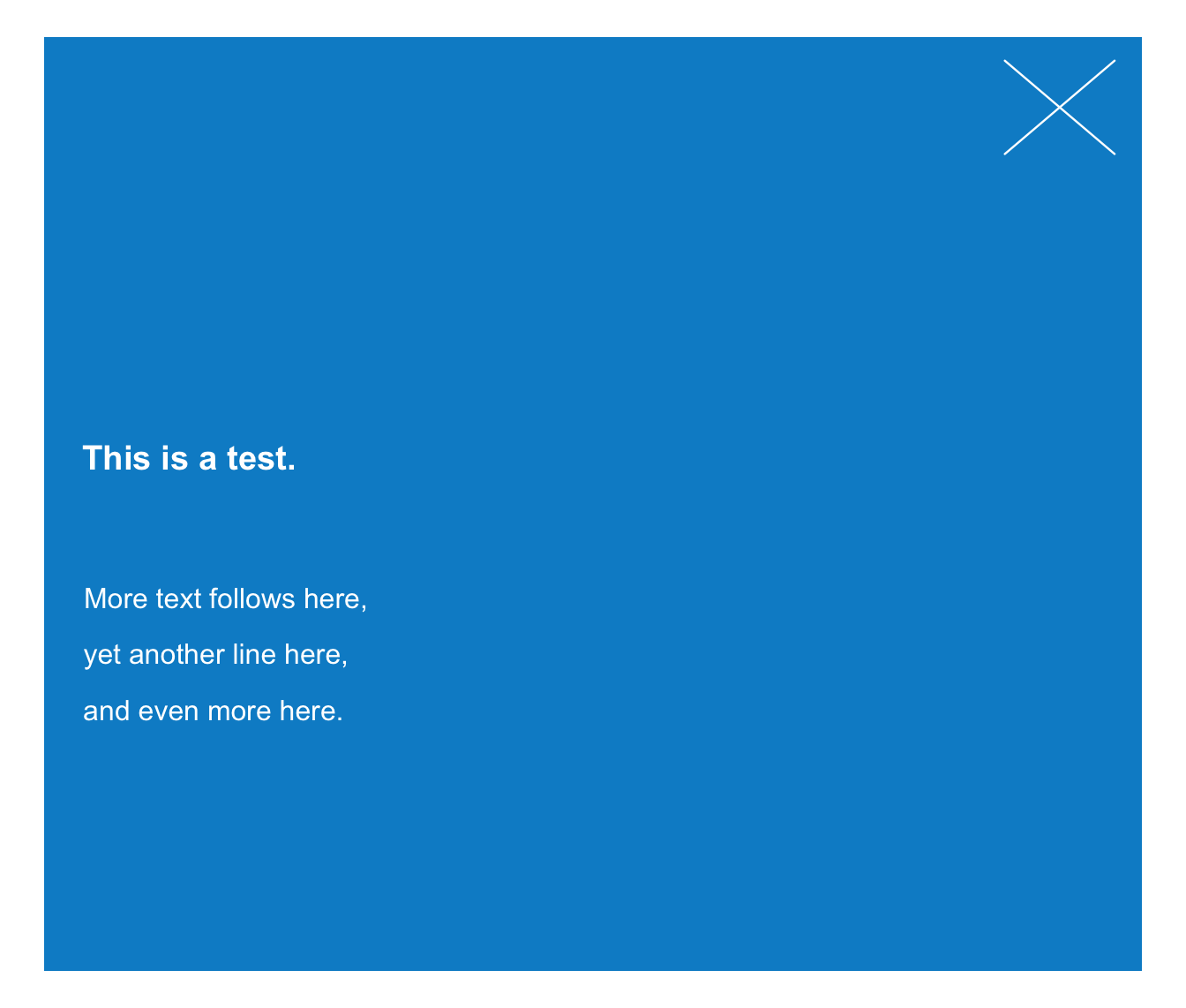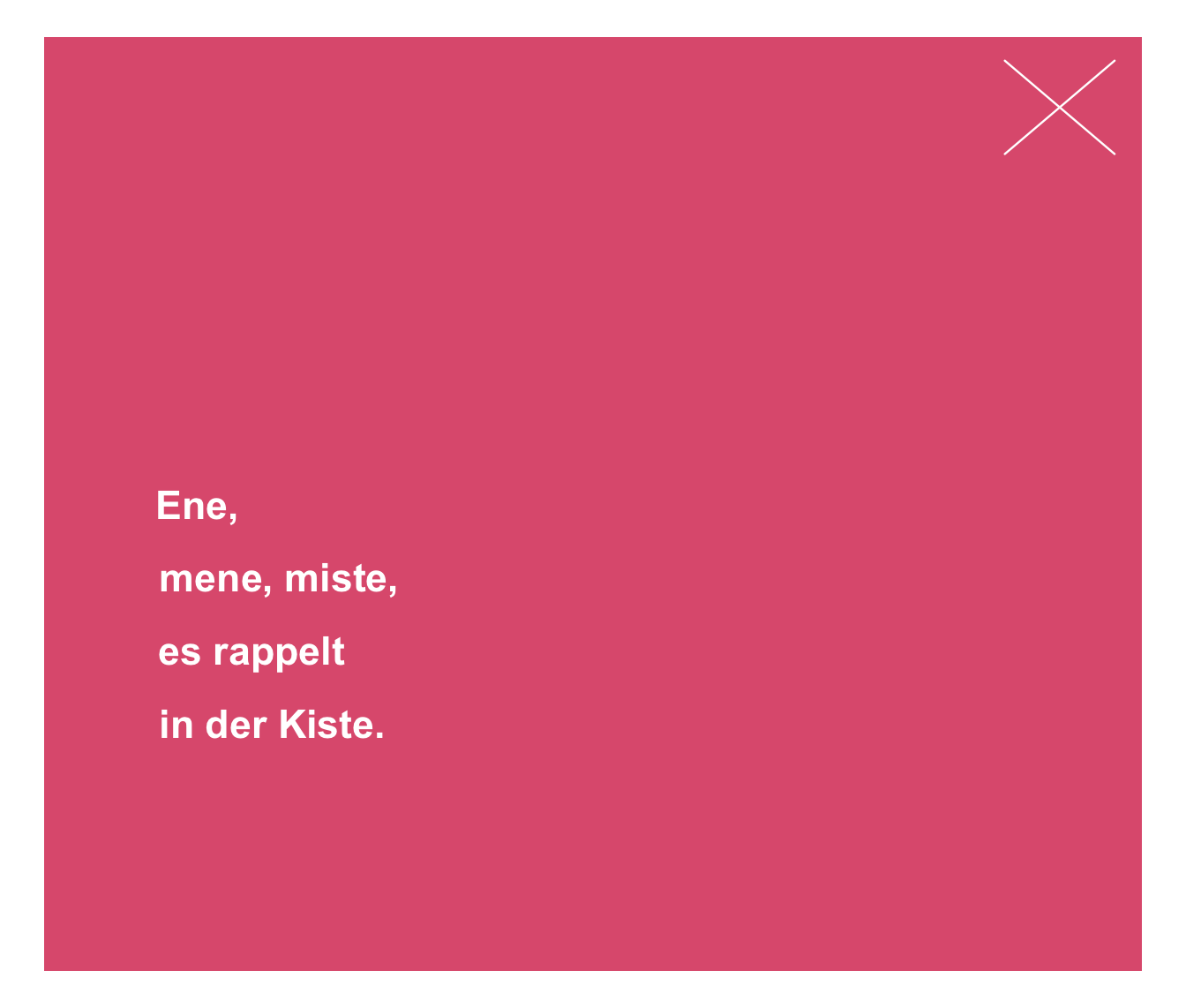post plots 1 or more text strings (provided as a character vector labels) to an (existing or new) xbox.

post(
labels,
x = 0.03,
y = 0.55,
y_layout = "even",
col = "white",
col_bg = Seeblau,
cex = 1,
font = 1,
new_plot = "none"
)

Arguments

labels A character vector specifying the text labels to be plotted. A numeric vector of x-coordinates at which the text labels in labels should be written. If the lengths of x and y differ, the shorter one is recycled. Default: x = .03. A numeric vector of y-coordinates at which the text labels in labels should be written. If the lengths of x and y differ, the shorter one is recycled. Default: y = .55. A numeric value or vector for the vertical spacing of labels in labels. 2 special values are "even" (i.e., even distribution of labels across available y-space) and "flush" (i.e., no space between adjacent labels, or y_layout = 0). Default: y_layout = "even". The color(s) of the text label(s). Default: col_lbl = "white". The background color(s) of the xbox. Default: col_bg = Seeblau. Numeric character expansion factor(s), multiplied by par("cex") to yield the character size(s). Default: cex = 1.0. The font type(s) to be used. Default: font = 1 (i.e., plain text). Should a new plot be generated? Set to "xbox" to plot to a basic xbox (with square dimensions, i.e., dim = c(1, 1)). Default: new_plot = "none" (i.e., assumes a pre-existing xbox).

Details

The positions of the text elements in labels can be specified by providing their coordinates (as x and y arguments) or by providing an initial position and an y_layout (see below).

Text formatting parameters (like col, col_bg, cex, font) are recycled to match length(labels).

post uses the base graphics system graphics::.